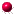XMM-Newton Science Analysis System

asmooth (asmooth-2.32.1) [xmmsas_20230412_1735-21.0.0]

## Ways of specifying the convolvers

In all the following descriptions, the convolving function is designated by, whereandare integer pixel numbers. Convolvers must necessarily be of finite extent, hence are only defined over a rectangular array.

It is necessary to have some way to determine the `phase' of any convolver: ie, which pixel of the convolver array should be taken to have row and column indicesandequal to zero. The rule applied by asmooth is as follows. If the convolver has for examplerows, then row 1 plus the integerpart ofis taken as the zeroth row. This means that ifis odd, the central row is the zeroth row, ie the row indices are taken to extend fromto; ifis even, the row indices run fromto. The same rule is applied to the columns.

Subsections

XMM-Newton SOC -- 2023-04-16